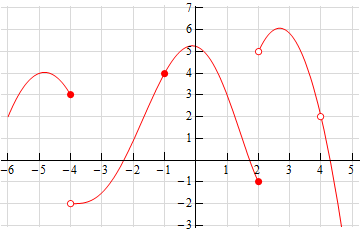Paul's Online Notes
Home / Calculus I / Limits / One-Sided Limits
Show Mobile Notice Show All Notes Hide All Notes
Mobile Notice
You appear to be on a device with a "narrow" screen width (i.e. you are probably on a mobile phone). Due to the nature of the mathematics on this site it is best views in landscape mode. If your device is not in landscape mode many of the equations will run off the side of your device (should be able to scroll to see them) and some of the menu items will be cut off due to the narrow screen width.

### Section 2.3 : One-Sided Limits

1. Below is the graph of $$f\left( x \right)$$. For each of the given points determine the value of $$f\left( a \right)$$, $$\mathop {\lim }\limits_{x \to {a^{\, - }}} f\left( x \right)$$, $$\mathop {\lim }\limits_{x \to {a^{\, + }}} f\left( x \right)$$, and $$\mathop {\lim }\limits_{x \to a} f\left( x \right)$$. If any of the quantities do not exist clearly explain why.

1. $$a = - 4$$
2. $$a = - 1$$
3. $$a = 2$$
4. $$a = 4$$Show All Solutions Hide All Solutions

a $$a = - 4$$ Show Solution

From the graph we can see that,

$\require{bbox} \bbox[2pt,border:1px solid black]{{f\left( { - 4} \right) = 3}}$

because the closed dot is at the value of $$y = 3$$.

We can also see that as we approach $$x = - 4$$ from the left the graph is approaching a value of 3 and as we approach from the right the graph is approaching a value of -2. Therefore, we get,

$\require{bbox} \bbox[4pt,border:1px solid black]{{\mathop {\lim }\limits_{x \to \, - {4^{\, - }}} f\left( x \right) = 3\hspace{0.25in}\hspace{0.25in}\& \hspace{0.25in}\mathop {\lim }\limits_{x \to \, - {4^{\, + }}} f\left( x \right) = - 2}}$

Now, because the two one-sided limits are different we know that,

$\require{bbox} \bbox[2pt,border:1px solid black]{{\mathop {\lim }\limits_{x \to \, - 4} f\left( x \right)\,\,{\mbox{does not exist}}}}$

b $$a = - 1$$ Show Solution

From the graph we can see that,

$\require{bbox} \bbox[2pt,border:1px solid black]{{f\left( { - 1} \right) = 4}}$

because the closed dot is at the value of $$y = 4$$.

We can also see that as we approach $$x = - 1$$ from both sides the graph is approaching the same value, 4, and so we get,

$\require{bbox} \bbox[4pt,border:1px solid black]{{\mathop {\lim }\limits_{x \to \, - {1^{\, - }}} f\left( x \right) = 4\hspace{0.25in}\hspace{0.25in}\& \hspace{0.25in}\mathop {\lim }\limits_{x \to \, - {1^{\, + }}} f\left( x \right) = 4}}$

The two one-sided limits are the same so we know,

$\require{bbox} \bbox[2pt,border:1px solid black]{{\mathop {\lim }\limits_{x \to \, - 1} f\left( x \right) = 4}}$

c $$a = 2$$ Show Solution

From the graph we can see that,

$\require{bbox} \bbox[2pt,border:1px solid black]{{f\left( 2 \right) = - 1}}$

because the closed dot is at the value of $$y = - 1$$.

We can also see that as we approach $$x = 2$$ from the left the graph is approaching a value of -1 and as we approach from the right the graph is approaching a value of 5. Therefore, we get,

$\require{bbox} \bbox[4pt,border:1px solid black]{{\mathop {\lim }\limits_{x \to \,{2^{\, - }}} f\left( x \right) = - 1\hspace{0.25in}\hspace{0.25in}\& \hspace{0.25in}\mathop {\lim }\limits_{x \to \,{2^{\, + }}} f\left( x \right) = 5}}$

Now, because the two one-sided limits are different we know that,

$\require{bbox} \bbox[2pt,border:1px solid black]{{\mathop {\lim }\limits_{x \to \,2} f\left( x \right)\,\,{\mbox{does not exist}}}}$

d $$a = 4$$ Show Solution

Because there is no closed dot for $$x = 4$$ we can see that,

$\require{bbox} \bbox[2pt,border:1px solid black]{{f\left( 4 \right)\,\,\,{\mbox{does not exist}}}}$

We can also see that as we approach $$x = 4$$ from both sides the graph is approaching the same value, 2, and so we get,

$\require{bbox} \bbox[4pt,border:1px solid black]{{\mathop {\lim }\limits_{x \to \,{4^{\, - }}} f\left( x \right) = 2\hspace{0.25in}\hspace{0.25in}\& \hspace{0.25in}\mathop {\lim }\limits_{x \to \,{4^{\, + }}} f\left( x \right) = 2}}$

The two one-sided limits are the same so we know,

$\require{bbox} \bbox[2pt,border:1px solid black]{{\mathop {\lim }\limits_{x \to \,4} f\left( x \right) = 2}}$

Always recall that the value of a limit (including one-sided limits) does not actually depend upon the value of the function at the point in question. The value of a limit only depends on the values of the function around the point in question. Therefore, even though the function doesn’t exist at this point the limit and one-sided limits can still have a value.